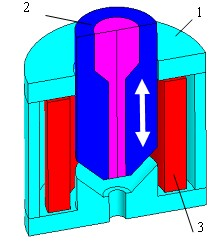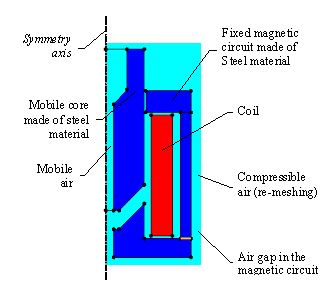# 2D example: Translating motion tutorial

## Foreword

This paragraph is a summary of cases treated in detail in the 2D example: "Translating motion tutorial".

The files relating to the studied cases, are accessible via the supervisor, Open Example context.

## Study carried out

The study proposed in the " Translating motion tutorial " is the study of the cylindrical electromagnet of an electro-valve (with conical air gap).

## Studied device

The studied device, represented on the figure below, includes the following elements:

• a magnetic circuit made up of two ferromagnetic parts (laminated):
• a fixed part
• a moving core (+ spring)
• a coil supplied by a 24-volt power supply## Modeled device

The device is modeled in Flux as represented below. Only one half of the device is describe in an axisymetric study domain.## Operation

The operation of this device is as follows:

The coil produces the magnetic flux necessary for the attraction of the moving part of the magnetic circuit.

The different phases of the process are as follows:

• the coil is supplied (voltage source)
• under the effect of the magnetic force, the upper part moves to make contact with the lower one; the electromagnet is closed

## Treated cases

The simulations are carried out in Flux by means of the study of two cases:

• case 1: study with the multi-static model (different linear positions)
• case 2: parametric study with the coupled load model

## Case 1

The first case is a study with the multi-static model (Magneto Static application):

In this first case ( multi-static model ), the moving part of the device can take various positions (fixed arbitrarily).

One is interested in the computation of the magnetic field for arbitrarily chosen positions of the core.

With this model it is possible to evaluate the force acting on the core at different positions.

## Case 2

The second case is a parametric study with the coupled load model (Transient Magnetic application):

In this second case ( coupled load model ), the moving part of the device is interdependent of a return spring whose constant of stiffness is a parameter of the problem.

One is interested in the dynamic behavior of the electromagnet and in the transient of the electric circuit.

The advantage of this model is to study the time variation of the position and speed of the core and the time variation of the electromagnetic force acting on the core for different values of the stiffness constant.